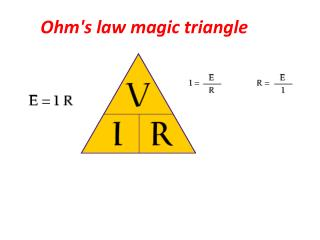DownloadDownload PresentationOhm's law magic triangle

# Ohm's law magic triangle

Télécharger la présentation## Ohm's law magic triangle

- - - - - - - - - - - - - - - - - - - - - - - - - - - E N D - - - - - - - - - - - - - - - - - - - - - - - - - - -
##### Presentation Transcript

1. Ohm's law magic triangle

2. Ohms law, defines the relationship between voltage, current and resistance. These basic electrical units apply to direct current, or alternating current. Ohm’s Law is the foundation of electronics and electricity. This formula is used extensively by electricians. Without a thorough understanding of “Ohm’s Law” an electrician can not design or troubleshoot even the simplest of electronic or electrical circuits. Ohm established in the late 1820’s that if a voltage was applied to a resistance then “current would flow and then power would be consumed”.

3. Voltage measured in volts, symbolized by the letters "E" or "V". Current measured in amps, symbolized by the letter "I". Resistance measured in ohms, symbolized by the letter "R".

4. If you know E and I, and wish to determine R, just eliminate R from the picture and see what's left:

5. If you know E and R, and wish to determine I, eliminate I and see what's left:

6. if you know I and R, and wish to determine E, eliminate E and see what's left:

7. Let's see how these equations might work to help us analyze simple circuits: If we know the values of any two of the three quantities (voltage, current, and resistance) in this circuit, we can use Ohm's Law to determine the third.

8. calculate the amount of current (I) in a circuit, given values of voltage (E) and resistance (R):

9. calculate the amount of resistance (R) in a circuit, given values of voltage (E) and current (I):

10. calculate the amount of voltage supplied by a battery, given values of current (I) and resistance (R):

11. Ohm’s Law power consumption through a resistance Some practical every day examples of this basic rule are: base board heaters, electric frying pans, toasters and electric light bulbs. The heater consumes power producing heat for warmth, the frying pan consumes power producing heat for general cooking, the toaster consumes power producing heat for cooking toast, and the electric light bulb consumes power producing heat and more important light. A further example is an electric hot water system. All are examples of Ohm’s Law.

12. The force or pressure behind electricity

13. milliamp or just mA

14. As a milliampere (milliamp or just mA) is 1/1000th of an ampere, we can convert mA to Amps by just dividing by 1000. Another way is to take the current in mA and move the decimal to the left three places to accomplish the division by 1000. Here's the scoop: 275 mA / 1000 = 0.275 Amps Note that the decimal in 275 is to the right of the 5, and it's written as 275.0 (with a 0 added to show where the decimal is). Moving the decimal to the left three places gets up to .275 Amps, but we usually hang a 0 in front of the decimal. To convert Amps to milliAmps, just multiply by 1000 or move the decimal to the right three places. Just the opposite of what we did here to convert the other way.